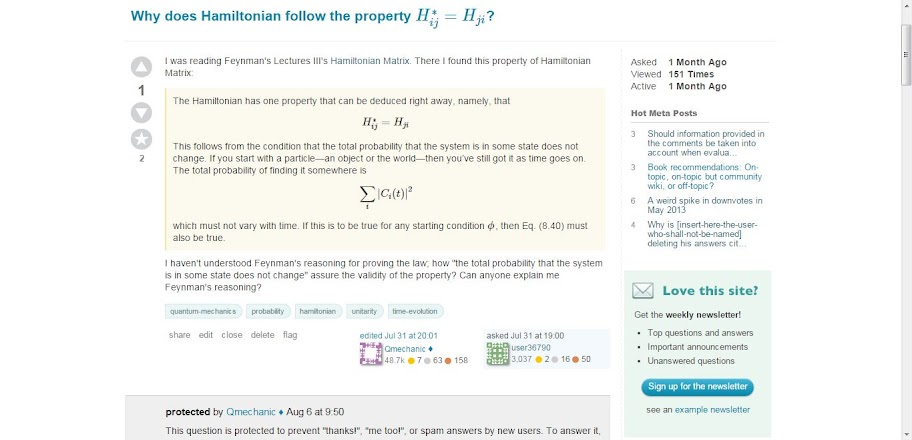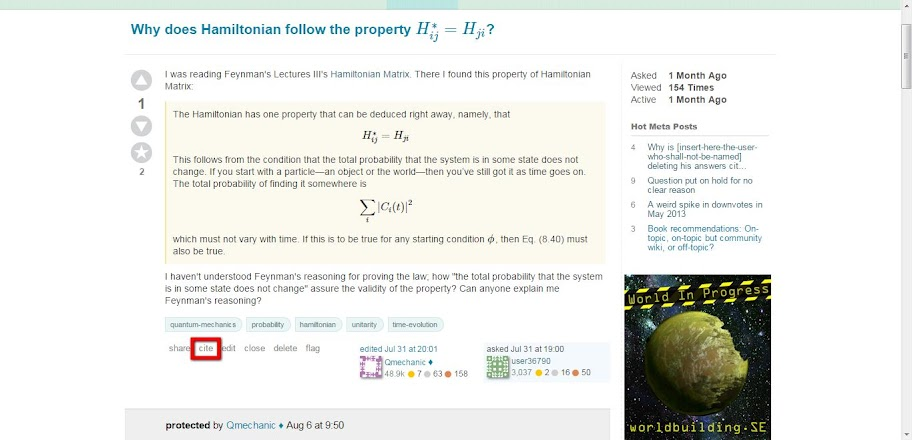# What is the function of cite below each question & answer?

I, today, saw one new thing below my question cite . I pressed it & this appeared:

Example citation:

user36790 (https://physics.stackexchange.com/users/36790/user36790), Why does Hamiltonian follow the property $H^*_{ij} = H_{ji}$?, URL (version: 2015-07-31): Why does Hamiltonian follow the property $H^*_{ij} = H_{ji}$?

BibTeX amsrefs

@MISC {197101, TITLE = {Why does Hamiltonian follow the property $H^*_{ij} = H_{ji}$?}, AUTHOR = {user36790 (https://physics.stackexchange.com/users/36790/user36790)}, HOWPUBLISHED = {Physics Stack Exchange}, NOTE = {URL:Why does Hamiltonian follow the property $H^*_{ij} = H_{ji}$? (version: 2015-07-31)}, EPRINT = {Why does Hamiltonian follow the property $H^*_{ij} = H_{ji}$?}, URL = {Why does Hamiltonian follow the property $H^*_{ij} = H_{ji}$?}

}

\bib{197101}{misc}{
title={Why does Hamiltonian follow the property $H^*_{ij} = H_{ji}$?},
author={user36790 (https://physics.stackexchange.com/users/36790/user36790)},
note={URL: Why does Hamiltonian follow the property $H^*_{ij} = H_{ji}$? (version: 2015-07-31)},
eprint={Why does Hamiltonian follow the property $H^*_{ij} = H_{ji}$?},
organization={Physics Stack Exchange}
}

Even yesterday I didn't see it. I really don't know what these mean; I am really ignorant of these stuffs.

Can anyone explain what this cite is actually meant for? What is its function?• How do you get the image of the site from the past? Oct 8, 2015 at 13:19
• @Ooker: What do you want to know?
– user36790
Oct 8, 2015 at 13:29
• i mean, if you are not aware that tomorrow the site is changed, then why do you take the image of it today, so that you can use it to ask tomorrow? Oct 8, 2015 at 13:37
• @Ooker: I love conspiracy theories! No, actually, you know about India? At most of the places, the internet speed is less than that of snail's pace; sometimes it becomes dead!! So, I generally don't close most of the pages I couldn't complete reading at the night & let them stay for the next morning or so without shutting down my laptop but rather letting it hibernate. This is the case here. I had the previous page & so I took the shot; that's it:)
– user36790
Oct 8, 2015 at 17:24

If you did this on a website, that's pretty easy to manually include the <a></a> tags and do what you need to do. However, if you are writing lecture notes, or a journal article, or a thesis, or something like that, it isn't always as easy. The cite button that you refer to gives you a citation in BibTeX format as well as a LaTeX bibitem format. One can use these when typesetting a document using LaTeX and a citation will occur where desired and the bibliography will contain the full information required by the license.